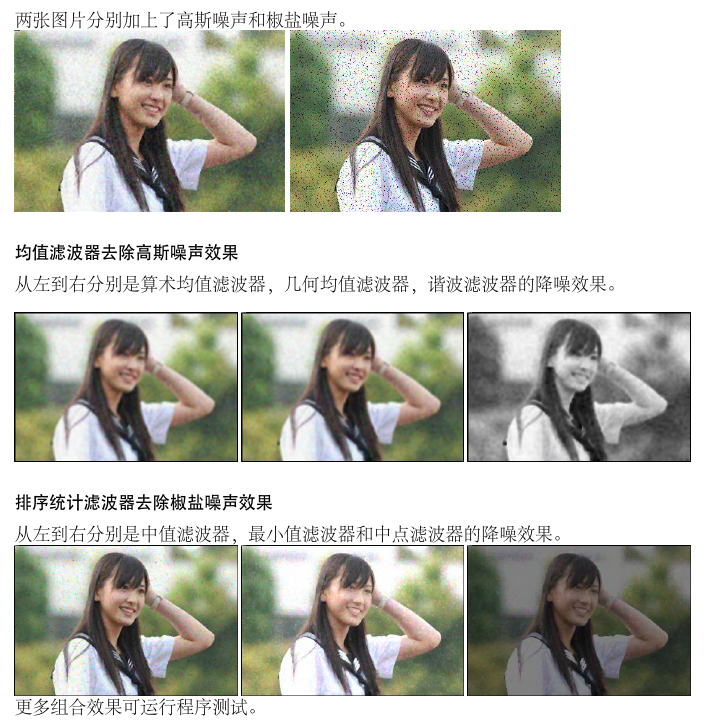# 一些简单的图像降噪算法

## 为什么要降噪

$p(z) = \frac{1}{\sqrt{2\pi}\sigma}exp[-\frac{(z-u)^{2}}{2\sigma^{2}}]$

## 算法实现

### 均值滤波器

$\hat{f}(x, y)=\frac{1}{mn}\sum\limits_{(p,q)\in W}g(p,q)$

$\hat{f}(x, y)=[ \prod\limits_{(p,q)\in W}g(p,q) ]^{\frac{1}{mn}}$

$\hat{f}(x, y)=\frac{mn}{\sum\limits_{(p,q)\in W}\frac{1}{g(p,q)}}$

### 排序统计滤波器

$\hat{f}(x, y)=median \ { g(p,q) }$

$\hat{f}(x, y)=(max\ or\ min){ g(p,q) }$

## 用Qt操作图像

Qt5把部分图像的操作封装成了一个类：QImage。每一张图片都可以抽象成一个QImage对象并用面向对象的方法进行操作。

Qt中，图像的显示需要依附于一个QLabel元素。由于我们的框架是Qt\ Widget，所以可以在当前的窗体（widget）中设置一个QLabel。 可以向QLabel元素在像素级导入一个QImage对象，然后通过show方法就能显示出图像。

## 算法测试结果